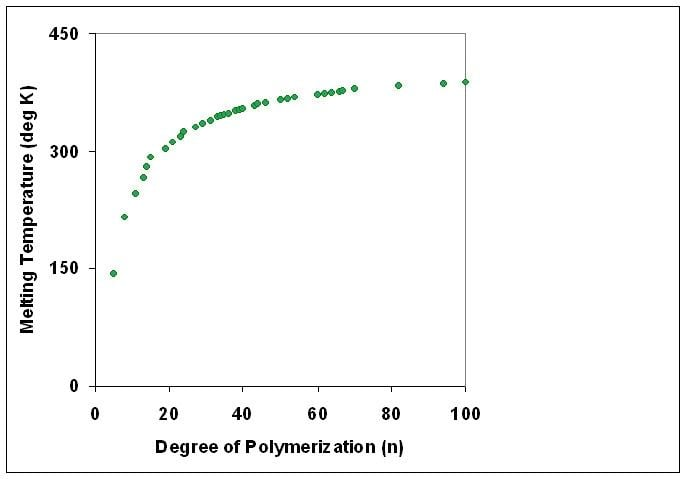# Degree of polymerization

Updated onThe degree of polymerization, or DP, is usually defined as the number of monomeric units in a macromolecule or polymer or oligomer molecule.

## Contents

For a homopolymer, there is only one type of monomeric unit and the number-average degree of polymerization is given by D P n X n = M n M 0 , where Mn is the number-average molecular weight and M0 is the molecular weight of the monomer unit. For most industrial purposes, degrees of polymerization in the thousands or tens of thousands are desired. This number does not reflect the variation in molecule size of the polymer that typically occurs, it only represents the mean number of monomeric units.

Some authors, however, define DP as the number of repeat units, where for copolymers the repeat unit may not be identical to the monomeric unit. For example, in nylon-6,6, the repeat unit contains the two monomeric units —NH(CH2)6NH— and —OC(CH2)4CO—, so that a chain of 1000 monomeric units corresponds to 500 repeat units. The degree of polymerization or chain length is then 1000 by the first (IUPAC) definition, but 500 by the second.

## Step-growth and chain-growth polymerization

In step-growth polymerization, in order to achieve a high degree of polymerization (and hence molecular weight), Xn, a high fractional monomer conversion, p, is required, according to Carothers' equation X ¯ n = 1 1 p For example, a monomer conversion of p = 99% would be required to achieve Xn = 100.

For chain-growth free radical polymerization, however, Carothers' equation does not apply. Instead long chains are formed from the beginning of the reaction. Long reaction times increase the polymer yield, but have little effect on the average molecular weight. The degree of polymerization is related to the kinetic chain length, which is the average number of monomer molecules polymerized per chain initiated. However it often differs from the kinetic chain length for several reasons:

• chain termination may occur wholly or partly by recombination of two chain radicals, which doubles the degree of polymerization
• chain transfer to monomer starts a new macromolecule for the same kinetic chain (of reaction steps), corresponding to a decrease of the degree of polymerization
• chain transfer to solvent or to another solute (a modifier or regulator also decreases the degree of polymerization
• ## Correlation with physical properties

Polymers with identical composition but different molecular weights may exhibit different physical properties. In general, increasing degree of polymerization correlates with higher melting temperature and higher mechanical strength.

## Number-average and weight-average

Synthetic polymers invariably consist of a mixture of macromolecular species with different degrees of polymerization and therefore of different molecular weights. There are different types of average polymer molecular weight, which can be measured in different experiments. The two most important are the number average (Xn) and the weight average (Xw).

The number-average degree of polymerization is a weighted mean of the degrees of polymerization of polymer species, weighted by the mole fractions (or the number of molecules) of the species. It is typically determined by measurements of the osmotic pressure of the polymer.

The weight-average degree of polymerization is a weighted mean of the degrees of polymerization, weighted by the weight fractions (or the number of molecules) of the species. It is typically determined by measurements of Rayleigh light scattering by the polymer.

Degree of polymerization Wikipedia